Last modified: 03/25/97

Acknowledgments

Much of this presentation is derived from the Wavelets Course given at SIGGRAPH 96. This course was organized by Peter Schröder of the California Institute of Technology and Wim Sweldens of Lucent Technologies Bell Laboratories.

The 3D surface material comes both from the above course notes and from the paper by Certain et. al. entitled, "Interactive Multiresolution Surface Viewing," presented at SIGGRAPH 96.

Table of Contents
Introduction

Wavelets, with their roots in signal processing and harmonic analysis, have had a significant impact in several areas of computer science. They have led to a number of efficient and easy to implement algorithms for use in such fields as:

• Image Compression and Processing;
• Global Illumination;
• Hierarchical Modeling;
• Animation;
• Volume Rendering and Processing;
• Multiresolution Painting;
• Image Query.
The development of wavelets has been motivated primarily by the need for fast algorithms to compute compact representations of functions and data sets. They exploit the structure (if any) in the data or underlying function, reorganizing the same in a hierarchical fashion.

The Haar Wavelet

The Haar Wavelet is probably the simplest wavelet to understand. Consider two numbers a and b, neighboring samples of a sequence. a and b have some correlation (hopefully) of which we'd like to take advantage. We use a simple linear transform to replace a and b by their average s and difference d.

s = (a + b) / 2
d = b - a
The aim is to reduce the number of bits required for d, which will be the case if a and b are highly correlated. This computation can be reversed to recover a and b:
a = s - d/2
b = s + d/2
This is the idea behind the Haar Wavelet. Consider a signal sn of 2n sample values. For each pair of values, we apply this average and difference transform. There are 2n-1 such pairs, producing that many average and difference values. We can think of the averages as a coarser resolution version of the original signal, and the differences as the higher resolution details. If the original signal is highly correlated, the coarse representation of the signal is very close to the original, and the details can be represented efficiently.

We can then apply the same average/difference transform to the coarser signal, splitting it into a yet coarser signal and more details. This transform can be applied until their is but a single signal value and a whole lot of detail values. The remaining signal is the average of the entire signal, and can be thought of as the DC or zero frequency of the original signal.

With some fancy footwork, this transform can be applied and reversed in place with no additional buffer space, and requires only O(N) time due to its hierarchical nature.

A 2D Wavelet Transform for Images

Progressive transmission of an image can benefit from a 2D wavelet transform as described here.

The process is conceptually really simple.  Given an image, we will create 4 new sub-images to replace it.  (Assume that the image size is 2n x 2n pixels.  This simplifies things a bit, as we will be able to symetrically subdivide the image down to single pixels.  But this requirement can be eliminated by scaling the image or enlarging it with 0's until it is 2n x 2n pixels.  After decoding the image, these pre-processing steps should be reversed.)

To create these four sub-images, we break the original image into 4 pixel blocks, 2 pixels to a side.  If the original image has size 2n x 2n, we should have 22n-2 blocks.  Now for each block, the top-right pixel will go directly into the top-right subimage.  The bottom-left pixel goes directly into the bottom-left subimage.  And the bottom-right pixel goes into the bottom-right subimage.  So these 3 subimages will look like a coarse version of the original, containing 1/4 of the original pixels.

The top-left pixel of each block does not go directly into the top-left subimage.  Rather, all 4 pixels of the block are averaged and placed into the top-left subimage.  So the top-left subimage is effectively a scaled down version of the original image at 1/4 the original size.  But it does not contain any of the original pixels itself (unless by chance).

This process can be repeated now for the top-left image.  We break it down into four new sub-images the same way, producing a top-left subimage of the previous top-left subimage that is now a scaled down image 1/16 the original size.  We repeat this process until our top-left subimage is only 1 pixel, with its color corresponding to the average of all pixels in the original image.

The newly encoded image lends itself to progressive transmission, as the top-left subimages can be transmitted first, yielding useful approximations, followed by the 3 corresponding subimages which contain original pixels to be used for reconstructing the next finer top-left subimage.  Reconstructing the 4 pixel blocks is simple.  One pixel is grabbed from each of the top-right, bottom-left, and bottom-right subimages.  Then the top-left original pixel is generated by taking the average value from the top-left subimage, multiplying it by 4, and subtracting out each of the other 3 original pixel values.  You are left with the original top-left value.  This is a lossless encoding.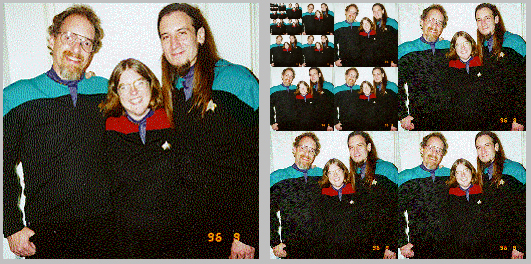I have written a simple Java applet that demonstrates this 2D wavelet transform.  Here lies the source code of the applet infrastructure and of the transform filter itself.

Multiresolution Surfaces

Much of this material is inaccessible without a sturdy background in multiresolution analysis. I will attempt to digest it somewhat and regurgitate it for the benefit of those not already initiated into this discourse community.

The wavelet approach to progressive transmission of 3D surfaces is basically the same as that used for curves, images, or any signal.  We must iteratively decompose the original, or high-resolution, surface into a low-resolution part and a detail part.  We end up with the coarsest representation of the original surface, along with a sequence of details which can be used to reconstruct the original.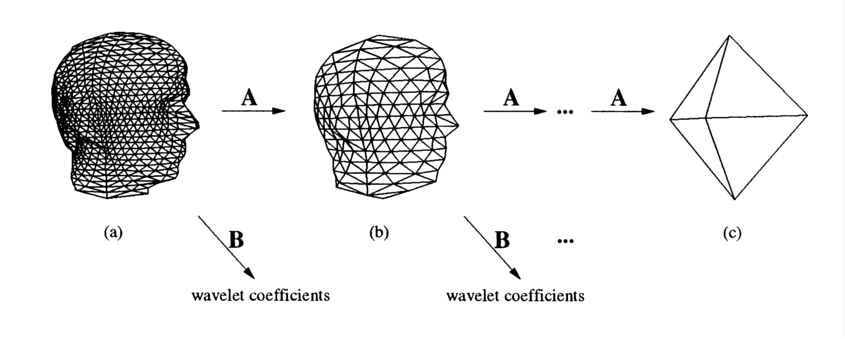The low-resolution part can be obtained by generating new vertex positions as the weighted averages of the original vertex positions.  As this is a linear operation, it can be expressed as multiplication by a matrix Aj.  Detail can likewise be expressed as multiplication by a matrix Bj.  This is recursively applied to the low-resolution part until the coarsest representation is obtained.

"Analysis" filters Aj and Bj can be inverted to produce "synthesis" filters Pj and Qj.  This synthesis can be viewed as two steps: splitting each face into 4 subtriangles by introducing new vertices at the midpoints of existing edges, and perturbing the new collection of vertices according to the wavelet coefficients.

A few goals guide the design of analysis and synthesis filters.  These are:

• We want the low-resolution versions to be good approximations of the original model;
• We want the magnitude of the wavelet coefficients to provide useful measures of error when equal to zero;
• We want the time complexity to grow linearly with the number of vertices.

Mathematical Framework

Here is where it begins to get dense.

Consider our coarsest representation of the model.  We'll call this the base mesh, or M0.  We create the next finer mesh, M1, by subdividing each triangular face into four new faces.  We do this by introducing new vertices at the midpoints of the parent face's three edges.  In turn, these new faces can again be subdivided to create M2.  The simplest base mesh is a tetrahedron, but this is arbitrary, and will be chosen depending on the topology of the original model.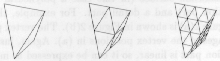These meshes are nested when considered in three space, as all points of M j are also points of M j+1.  The nested meshes are used to define a sequence of nested spaces. With each mesh M j, we define V j as the set of all continuous functions that are linear on each face of M j.  These spaces are nested since any function linear on the faces of M j is also linear on the faces of M j+1.  Each function in V j maps points of M0 into a real number:

V j := {f | f : M0 --> R and f linear on faces of M j}
Now for some scaling functions {ø ji(x)}i that span V j.  Since V j contains only piecewise linear functions, a member of V j is uniquely determined by its values at the vertices of M j.  So a natural basis would consist of the so-called "hat" functions.  At every vertex i of M j is the unique member ø ji(x) in V j that achieves value 1 at vertex i and zero at all other vertices of M j.  Wavelets w ji(x) are simply basis functions for complement spaces W j = V j+1 - V j.

These hat functions can be used to construct polyhedral surfaces in 3-space: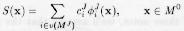This defines a polyhedron with vertex positions cJi = (xJi,yJi,zJi) that is topologically equivalent to the base mesh M0.  The vertices of S have the connectivity of MJ, or the connectivity of M0 recursively subdivided J times.  This sort of mesh is said to have subdivision connectivity.

Expressing S(x) in wavelet form looks like this: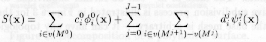for chosen wavelets wJi(x).  Now we must construct such wavelets.

k-disc Wavelets

We will construct a biorthogonal wavelet basis for polyhedral surfaces.  It will have the following properties:

• Analysis and synthesis can both be accomplished in linear time.
• The wavelets are "nearly orthogonal" to the scaling functions, meaning that low-resolution surface approximations are close to least-squares best.
We start with lazy wavelets, which for polyhedral surfaces consist of the scaling functions (i.e., the hat functions) in V j+1 centered on the midpoints of M j.  Although very simple, lazy wavelets are far from orthogonal to members of V j, meaning that the coarse versions of a full-resolution surface are far from least-squares best.  Fortunately, lifting can be used to make them "more orthogonal," resulting in k-disk wavelets.

Consider a vertex i of M j+1 located at the midpoint of an edge e of M j.  The k-disk wavelet centered at vertex i is a function of the formwhere Nk is a set of vertices of M j in a neighborhood of vertex i.  The neighborhoods are defined recursively: The neighborhood N0 for the 0-disk wavelet consists of the endpoints of e; Nk contains the vertices of all triangles incident on Nk-1.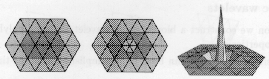The coefficients s jiv are chosen to minimize the norm of the orthogonal projection of wJi onto V j.  They are determined by solving the following system of linear equations: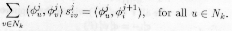As is usual in wavelet analysis, we will use a filterbank procedure that splits a mesh S j+1(x) described by vertex positions c j+1i into a low-resolution part S j(x), with vertex positions c ji, and a detail part described by wavelet coefficients d ji.  This decomposition is particularly simple for k-disk wavelets.
Let u and v be vertices of M j, and let i be their midpoint.  The wavelet wJi is therefore centered at i.  Then the wavelet coefficient can be computed according to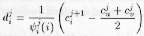Once all wavelet coefficients have been computed at level j, S j(x) can be determined by subtracting out their collective contribution, since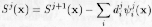Back to the Real World

Now that we are out of the woods mathematically, we can use these techniques to transform an arbitrary mesh made up of vertices, edges, and faces into a representation that is suitable for progressive transmission.

The techniques described above require a base mesh, M0, and a parameterized description of the mesh.  All we have is the original full-resolution mesh, M.  Fortunately, we have at our disposal the remeshing algorithm of Eck et. al., which, given an arbitrary mesh as input, will output a base mesh with relatively few faces, and a parameterization of the surface.  Just what we need.

We can then apply the k-disk analysis described above.  Once we have the wavelet coefficients, we sort them in order of magnitude.  After transmitting the base mesh, we send the most influencial coefficients first, as this will result in a quicker convergence upon the full-resolution model.

As described by Certain et. al., vertex color data can be represented by wavelet coefficients in much the same way as vertex position data is.

See the SIGGRAPH 96 Visual Proceedings for a demonstration of these techniques.  In addition to progressive transmission, the techniques are equally important for surface and texture compression, continuous level-of-detail control, and multiresolution editing.

References and Related Links

Copyright © 1997 by Benj Lipchak.
All rights reserved.